# Concentric circles

There is given a circle K with a radius r = 8 cm. How large must a radius have a smaller concentric circle that divides the circle K into two parts with the same area?

Correct result:

r2 =  5.6569 cm

#### Solution: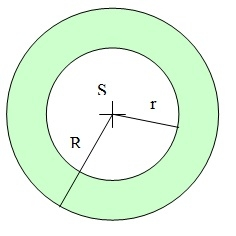We would be pleased if you find an error in the word problem, spelling mistakes, or inaccuracies and send it to us. Thank you!#### You need to know the following knowledge to solve this word math problem:

We encourage you to watch this tutorial video on this math problem:

## Next similar math problems:

• Maxwell’s inductance bridge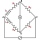The four arms of Maxwell’s inductance bridge are; Arm AB contains an Inductive coil of inductance L1 having resistance R1. Arms BC and CD contain non-inductive resistances of 200Ω and 100Ω respectively. Arm AD contains standard inductor of inductance L2 a
• The projectileThe projectile was fired horizontally from a height of h = 25 meters above the ground at a speed of v0 = 250 m/s. Find the range and flight time of the projectile.
• As shownAs shown, in △ ABC, ∠C = 90°, AD bisects ∠BAC, DE⊥AB to E, BE = 2, BC = 6, then the perimeter of △ BDE
• What are 2What are the two more terms of the GP a, ax, ax2, ax3, __, __?
• Find the 19Find the 1st term of the GP ___, -6, 18, -54.
• If the 3If the 6th term of a GP is 4 and the 10th is 4/81, find common ratio r.
• Up and down motionWe throw the body from a height h = 5 m above the Earth vertically upwards v0 = 10 m/s. How long before we have to let the second body fall freely from the same height to hit the Earth at the same time?
• Sum of GP members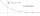Determine the sum of the GP 30, 6, 1.2, to 5 terms. What is the sum of all terms (to infinity)?
• Acute triangle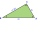In the acute triangle KLM, V is the intersection of its heights and X is the heel of height to the side KL. The axis of the angle XVL is parallel to the side LM and the angle MKL is 70°. What size are the KLM and KML angles?
• Telco company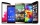The upstairs communications company offers customers a special long distance calling rate that includes a \$0.10 per minute charge. Which of the following represents this fee scheduale where m represents the number of minutes and c is the overall cost of t
• Trapezoid 25Trapezoid PART with AR||PT has (angle P=x) and (angle A=2x) . In addition, PA = AR = RT = s. Find the length of the median of Trapezoid PART in terms of s.
• Sphere cutA sphere segment is cut off from a sphere k with radius r = 1. The volume of the sphere inscribed in this segment is equal to 1/6 of the volume of the segment. What is the distance of the cutting plane from the center of the sphere?
• What is 10What is the 5th term, if the 8th term is 80 and common ratio r =1/2?
• Volume per timeHow long does fill take for a pump with a volume flow of 200 l per minute fill a cube-shaped tank up to 75% of its height if the length of the cube edge is 4 m?
• GardensThe area of the square garden is 3/4 of the area of the triangular garden with sides of 80 m, 50 m, 50 m. How many meters of the fence do we need to fence a square garden?
• Space diagonal anglesCalculate the angle between the body diagonal and the side edge c of the block with dimensions: a = 28cm, b = 45cm and c = 73cm. Then, find the angle between the body diagonal and the plane of the base ABCD.How long is a ladder that touches on a wall 4 meters high and its lower part is 3 meters away from the wall?